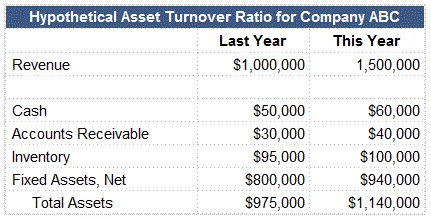## What is the Asset Turnover Ratio?

The asset turnover ratio is a measure of how efficiently a company's assets generate revenue. It measures the number of dollars of revenue generated by one dollar of the company's assets.

## How Does the Asset Turnover Ratio Work?

The formula for the turnover ratio is: Revenue / Average Total Assets

Let's look at an example using the following hypothetical information for Company ABC:Revenue is found on the income statement, and total assets are found on the balance sheet.

Using the asset turnover ratio formula and the information above, we can calculate that Company ABC's asset turnover ratio this year was:

\$1,500,000 / [(\$975,000 + \$1,140,000)/2] = 1.418

This means that for every dollar of Company ABC's assets, Company ABC generated \$1.42 in revenue.

It is important to understand that the age of a company's assets can lead to different asset turnover ratios for similar companies. A company with older assets (which have higher accumulated depreciation and hence lower book values) might have a higher asset turnover ratio than a company with the same revenues but newer, higher-book value assets.

## Why Does the Asset Turnover Ratio Matter?

In general, a low asset turnover ratio suggests problems with excess production capacity, poor inventory management, or lax collection methods. Increases in the asset turnover ratio over time may indicate a company is 'growing into' its capacity (while a decreasing ratio may indicate the opposite), but remember that asset purchases made in anticipation of coming growth (or the sale of unnecessary assets in anticipation of declining growth) can suddenly and somewhat artificially change a company's asset turnover ratio.

Low-margin industries tend to have higher asset turnover ratios than high-margin industries because low-margin industries must offset lower per-unit profits with higher unit-sales volume. Additionally, capital-intensive companies will typically have lower asset turnover ratios than companies using fewer assets. This is why comparison of asset turnover ratios is generally most meaningful among companies within the same industry, and the definition of a 'high' or 'low' ratio should be made within this context.